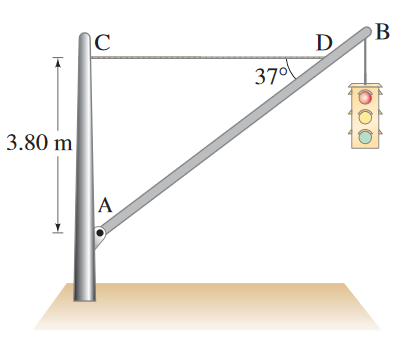Raúl's web!

# Problem 19 (hard problem)

A traffic light hangs from a pole. The uniform aluminium pole AB is (l)m long and has a (m)kg. The mass of the traffic light is (M)kg. Determine the tension (FT/FAX)newtons in the horizontal massless cable CD, and the vertical and horizontal components of the force exerted by the pivot A on the aluminium pole (FA)newtons.

a)Please enter the following data to calculate the previous equation:

 Enter the height (h). Enter the distance (l). Enter the angle (α). Enter the small mass (m). Enter the big mass (M). Equation 1 result is Comment:

b)

Please enter the following data to calculate the previous equation:

 Enter the small mass (that you used in the last equation).. Enter the big mass (that you used in the last equation). Equation 2 result is Comment:

Please enter the following data to calculate the previous equation:

 Enter the resultant force from equation 1 (FAX). Enter the resultant force from equation 2 (FAY). Equation 3 result is Comment: